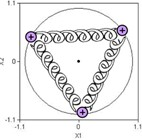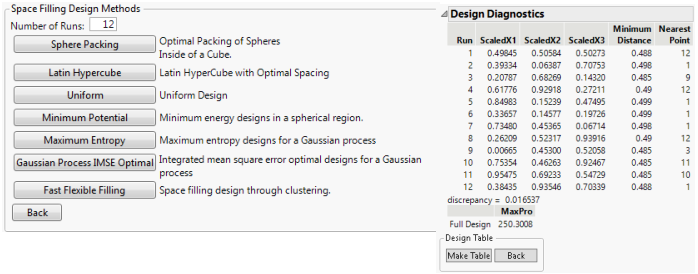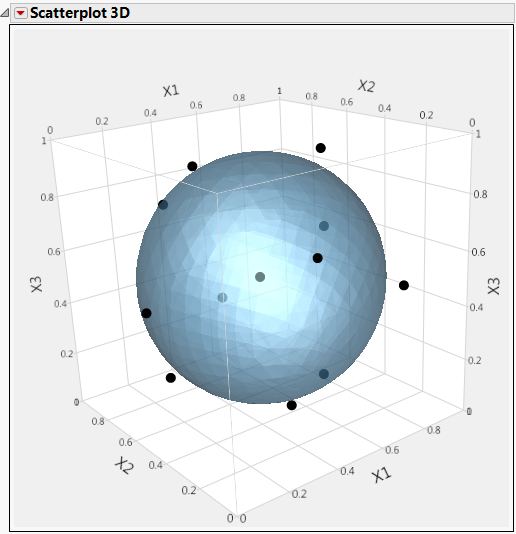Design of Experiments Guide > Space-Filling Designs > Minimum Potential Designs
Publication date: 11/10/2021

# Minimum Potential Designs

The Minimum Potential design spreads points out inside a sphere. To understand how this design is created, imagine the points as electrons with springs attached to every other point, as illustrated in Figure 21.17. The coulomb force pushes the points apart, but the springs pull them together. The design is the spacing of points that minimizes the potential energy of the system.

Figure 21.17 Minimum Potential DesignMinimum Potential designs:

have spherical symmetry

are nearly orthogonal

have uniform spacing

To construct a Minimum Potential example design:

1. Select DOE > Special Purpose > Space Filling Design.

See Factors.

3. Alter the factor level values to 0 and 1.

4. Click Continue.

5. In the design specification dialog (shown on the left in Figure 21.18), enter a sample size (Number of Runs). This example uses a sample size of 12.

6. Click the Minimum Potential button. JMP creates this design and displays the design runs and the design diagnostics (shown on the right in Figure 21.18).

Figure 21.18 Space-Filling Methods and Design Diagnostics for Minimum Potential Design7. Click Make Table.

You can see the spherical symmetry of the Minimum Potential design using the Scatterplot 3D graphics platform.

1. After you make the JMP design table, choose the Graph > Scatterplot 3D command.

2. In the Scatterplot 3D launch dialog, select X1, X2, and X3 as Y, Columns and click OK to see the initial three-dimensional scatterplot of the design points.

3. To see the results similar to those in Figure 21.19:

Click the Scatterplot 3D red triangle and select the Normal Contour Ellipsoids.

Right-click the plot and select Settings, and then increase the marker size using the Marker Size slider.

Now the even spread of the points on the surface of the ellipsoid can be visualized.

Figure 21.19 Minimum Potential Design Points on Sphere